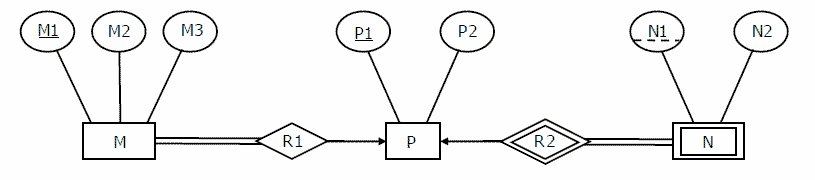Related Articles
GATE | GATE CS 2008 | Question 85
• Difficulty Level : Easy
• Last Updated : 07 Sep, 2018

Consider the following ER diagram.The minimum number of tables needed to represent M, N, P, R1, R2 is
(A) 2
(B) 3
(C) 4
(D) 5

Answer: (B)

Explanation: Answer is B, i.e, 3 minimum tables.

M, P are strong entities hence they must be represented by separate tables.

Many-to-one and one-to-many relationship sets that are total on the many-side can be represented by adding an extra attribute to the “many” side, containing the primary key of the “one” side. ( This way no extra table will be needed for Relationship sets )

M table is modified to include primary key of P side(i.e. P1). N is weak entity, and is modified to include primary key of P (i.e, P1).

Therefore there would be minimum of 3 tables with schema given below :

```M ( M1, M2, M3, P1)
P ( P1, P2 )
N ( P1, N1, N2 )```My Personal Notes arrow_drop_up